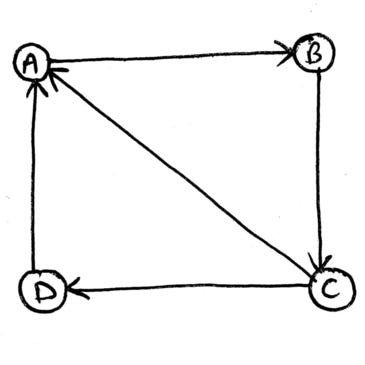Given two nodes for a given directed graph, find if there is a route between them.

Let us take a graph for example. And let the two nodes be A and C.

First let us check if there is a direct path between A and C. If yes, the problem was simple. But if not, we will try to find if there is a third node through which we can go from A to C.We can see that there isn’t any direct path from A to C, but there is a path from A to B and from B to C. Therefore, there is a route from A to C.

To find a route from A to C, we will have to traverse all the nodes starting from A and ending at C.

For graph traversal, we can use the Depth First Search Algorithm.

The Depth First Search Algorithm works in the following manner -

1. Take a Stack, and push the source node into it.
2. Find all the neighbors of the just pushed node and push them into the stack.
3. Come to the next element of the stack and push all the neighbors of this node.
4. Repeat the above steps until the end of the stack.
5. If during the above process at some point in time we encounter the destination node, we finally get a route from source to destination.

The below-given code is in the C programming language.

```#include<stdio.h>
#include<stdbool.h>

int top = -1;

void push(int stk, int x){
top = top + 1;
stk[top] = x;
}

bool presentInStack(int stk, int x){
int a;
for(a = 0 ; a < top+1 ; a++){
if(stk[a] == x){
return true;
}
}
return false;
}

void route(int arr, int x, int m, int n){
int a, i, stk;
push(stk,x);
for(i = 0 ; i <= top ; i++){
x = stk[i];
for(a = 0 ; a < n ; a++){
if(arr[x-1][a] == 1){
if(a + 1 == m){
printf("Yes! There's a route.\n");
return;
}
if(presentInStack(stk, a+1) == false){
push(stk,a+1);
}
}
}
}
printf("No! There isn't any route.\n");
}

void main(){
int arr = {
{0,1,0,1},
{0,0,1,0},
{1,0,0,1},
{0,0,0,0}
};

route(arr,1,3,4);
}
```